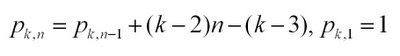## Monday, August 11, 2008

### Polygonal Number FormulasA few of the posts on this blog have dealt with the polygonal numbers. Polygonal numbers are a mainstay of recreational and school mathematics, providing a nice bridge between numbers and shapes. The diagrams above (created using the instructions in an earlier post) show some of the hexagonal numbers.

This post is intended to provide some (limited) background information on two-dimensional polygonal numbers. A previous post suggested an approach for generating a formula for higher-dimensional polygonal numbers. Future posts will elaborate on these numbers some more.

Some examples of two-dimensional polygonal numbers are:

the triangular numbers: 1, 3, 6, 10, 15, ...
the square numbers: 1, 4, 9, 16, 25, ...
the pentagonal numbers: 1, 5, 12, 22, 35,...
the hexagonal numbers: 1, 6, 15, 28, 45, ...

Comparing the listing for the hexagonal numbers with the diagrams above, you can see how the sequences are built diagrammatically. In general, beginning with a single dot, k-sided polygons are built by adding layers (called gnomons) consisting of k-2 segments, with each segment of the gnomon having one more dot than the segments of the previous layer. In this way, the nth gnomon consists of segments each n dots long, but with k-3 dots shared by adjoining segments (the corners).

The description above can lead you to a recursive formula for k-polygonals, writing p_k,n for the nth k-polygonal number:Unwinding the recursion gives you a summation formula for k-polygonals:Knowing a little about sums gives you the direct formula for k-polygonals:Coming a little out of left-field is this combinatorial formula for k-polygonals:This last formula expresses two ideas: that the triangular numbers correspond to the r=0 column of Pascal's triangle, and that every polygonal number can be "triangulated":The combinatorial formula for p_kn can be generalized to higher-dimensional polygonal numbers (pyrimidal numbers, etc.). See this previous post for some discussion on this.

The recreation here lies in showing that the various formulas for p_k,n are really the same, and then exploring the relationships between the different k-polygonals. A great resource is J.H. Conway and R.K. Guy's The Book of Numbers.